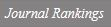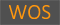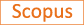Higher Hickerson formula

Title
Higher Hickerson formula
Authors
Lee J.Jun B.Chae H.-J.
Ewha Authors
SCOPUS Author ID
이정연Issue Date
2017
Journal Title
Journal of Number Theory
ISSN
0022-314XCitation
Journal of Number Theory vol. 170, pp. 191 - 210
Keywords
Generalized Dedekind sumsMeyer's formulaPartial zeta functionReal quadratic fieldsSiegel's formula
Publisher
Indexed
SCI; SCIE; SCOPUSDocument Type
Article
Abstract
In , Hickerson made an explicit formula for Dedekind sums s(p,q) in terms of the continued fraction of p/q. We develop analogous formula for generalized Dedekind sums si,j(p,q) defined in association with the xiyj-coefficient of the Todd power series of the lattice cone in R2 generated by (1,0) and (p,q). The formula generalizes Hickerson's original one and reduces to Hickerson's for i=j=1. In the formula, generalized Dedekind sums are divided into two parts: the integral sij I(p,q) and the fractional sij R(p,q). We apply the formula to Siegel's formula for partial zeta values at a negative integer and obtain a new expression which involves only sij I(p,q) the integral part of generalized Dedekind sums. This formula directly generalizes Meyer's formula for the special value at 0. Using our formula, we present the table of the partial zeta value at s=−1 and −2 in more explicit form. Finally, we present another application on the equidistribution property of the fractional parts of the graph (pq,Ri+jqi+j−2sij(p,q)) for a certain integer Ri+j depending on i+j. © 2016 Elsevier Inc.
DOI
10.1016/j.jnt.2016.06.003
Appears in Collections:
연구기관 > 수리과학연구소 > Journal papers
Files in This Item:
There are no files associated with this item.
Export
RIS (EndNote)
XLS (Excel)
XML